Study P5 Mathematics Decimals - Operations & Conversions - Geniebook# Decimals - Operations & Conversions

In this article we will learn about Decimals. We will study the various operations and the conversions of decimals into fractions and vice versa. The details covered are as per the Primary 5 Math requirements.

The lesson objectives are:

1. Multiplying by $$10$$$$100$$ or $$1000$$
2. Multiplying by multiples of $$10$$$$100$$ or $$1000$$
3. Dividing by $$10$$$$100$$ or $$1000$$
4. Dividing by multiples of  $$10$$$$100$$ or $$1000$$
5. Conversion of measurements

## Conversion of fractions to decimals and vice-versa

Question 1:

Express \begin{align} 1\frac {3}{5} \end{align} as decimal.

Solution:

\begin {align*} 1\frac {3}{5} &= 1\frac {3}{5} \\ &= 1.6 \end {align*}

$$1.6$$

Question 2:

Express \begin{align} 2\frac {1}{4} \end{align} as decimal.

Solution:

\begin {align*} 2\frac {1} {4} &= 2\frac {25}{100} \\ &= 2.25 \end {align*}

$$2.25$$

Question 3:

Express \begin{align} 3\frac {3}{8} \end{align} as a decimal.

Solution:

\begin {align*} 3 \frac {3} {8} &= 3 \frac {375}{1000} \\ &= 3.375 \end {align*}

$$3.375$$

Question 4:

Express \begin{align} 2\frac {5}{9} \end{align} as a decimal rounded to $$2$$ decimal places.

Solution:\begin {align*} \frac {5} {9} &≈ 0.555 \\ &≈ 0.56 &\text{(2 decimal places)} \\ \\ 2 \frac {5} {9} &= 2+ \frac {5} {9} \\ &≈ 2+0.56\\ &≈ 2.56 &\text{(2 decimal places)} \end {align*}

$$2.56$$

Question 5:

Express $$1.4$$ as a fraction in its simplest form.

Solution:

\begin {align*} 1.4 &= 1 \frac {4}{10} \\ &= 1 \frac {2}{5} \end {align*}

\begin{align} 1 \frac {2}{5} \end{align}

Question 6:

Express $$2.12$$ as a fraction in its simplest form.

Solution:

\begin {align*} 2.12 &= 2 \frac {12}{100} \\ &= 2 \frac {3}{25} \end {align*}

\begin{align} 2 \frac {3}{25} \end{align}

Question 7:

Express $$3.625$$ as a fraction in its simplest form.

Solution:

\begin {align*} 3.625 &= 3 \frac {625}{1000} \\ &= 3 \frac {5}{8} \end {align*}

\begin{align} 3 \frac {5}{8} \end{align}

## 1. Multiplying by $$10$$, $$100$$ or $$1000$$

Tip: When a decimal is multiplied by $$10$$$$100$$ or $$1000$$, the decimal point shifts to the right by the number of ‘$$0$$’s.

Example:Question 1:

Multiply $$5.9$$ by $$10$$

Solution: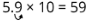$$59$$

Question 2:

Multiply $$0.404$$ by $$100$$

Solution: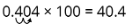$$40.4$$

Question 3:

Multiply $$76.9$$ by $$1000$$

Solution: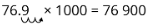$$76 \;900$$

## 2. Multiplying by multiples of  $$10$$, $$100$$ or $$1000$$

Examples:Question 1:

Multiply $$0.05$$ by $$60.$$

Solution: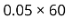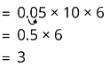$$3$$

Question 2:

Multiply $$3.64$$ by $$300.$$

Solution: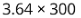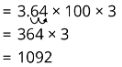$$1092$$

Question 3:

Multiply $$2.617$$ by $$7000$$.

Solution: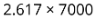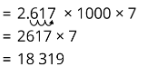$$18 \;319$$

## 3. Dividing by $$10$$, $$100$$ or $$1000$$

Tip: When a decimal is divided by $$10$$$$100$$ or $$1000$$ the decimal point shifts to the left by the number of ‘$$0$$’s.

Examples:Question 1:

Divide $$5.8$$ by $$10$$

Solution: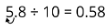$$0.58$$

Question 2:

Divide $$30.3$$ by $$100$$

Solution: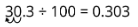$$0.303$$

Question 3:

Divide $$2$$ by $$1000$$.

Solution: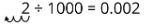$$0.002$$

## 4. Dividing by multiples of $$10$$, $$100$$ or $$1000$$

Examples:Question 1:

Divide $$2.1$$ by $$30$$

Solution: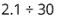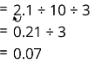$$0.07$$

Question 2:

Divide $$763$$ by $$700$$

Solution: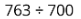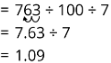$$1.09$$

Question 3:

Divide $$535$$ by $$5000$$.

Solution: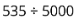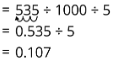$$0.107$$

## 5. Conversion of measurements

 \begin{align} 1 \;km &= 1000 \;m \\ 1 \;m &= 100 \;cm \end{align}\begin{align} 1 \;kg &= 1000 \;gm \end{align}\begin{align} 1 \;\ell &= 1000 \;m\ell \\ \end{align}When we convert from a larger unit to a smaller unit, we multiply.

Question 1:

Express $$0.77 \;km$$ in metres.

Solution:

\begin{align} 0.77 \;km &= 0.77 \times 1000 \;m \\ &= 770 \;m \end{align}

$$770 \;m$$

Question 2:

Express $$12.05 \;kg$$ in kilograms and grams.

Solution:

\begin{align} 12.05 \;kg &= 12.05 × 1000 \;g \\ &= 12 \;050 \;g \\ &= 12 \;kg \;50 \;g \end{align}

$$12 \;kg \;\;50 \;g$$

Question 3:

Express \begin{align}​ 4 \frac {1}{25} \;m \end{align} in metres and centimetres.

Solution:

\begin{align}​ 4 \frac {1}{25} \; m &= 4 \frac {4}{100} \;m \\ &= 4.04 \;m \\ &= 4.04 \times 100 \;m \\ &= 404 \;cm \\ &= 4 \;m \;4 \;cm \end{align}

$$4 \;m \;4 \;cm$$

Note: When we convert from a smaller unit to a larger unit, we divide.

Question 4:

Express $$86 \;m\ell$$ in litres.

Solution:

$$86 \;m\ell \div 1000 = 0.086 \;\ell$$

$$0.086 \;\ell$$

Question 5:

Express $$2 \;m \;5 \;cm$$ in metres.

Solution:

\begin{align}​ 2 \;m \;5 \;cm &= 205 \;cm \\ &= 205 \div 100 \\ &= 2.05 \div m \end{align}

$$2.05 \;m$$

Continue Learning
Volume Of A Liquid Decimals - Operations & Conversions
Ratio: Introduction Average - Formula
Percentage, Fractions And Decimals Whole Numbers
Strategy - Equal Stage Angle Properties
Table Rates Whole Number Strategy: Gap & Difference
Fractions - Addition & Subtraction Ratio Strategy: Repeated IdentityPrimaryPrimary 1Primary 2Primary 3Primary 4Primary 5EnglishMaths
Volume Of A Liquid
Decimals - Operations & Conversions
Ratio: Introduction
Average - Formula
Percentage, Fractions And Decimals
Whole Numbers
Strategy - Equal Stage
Angle Properties
Table Rates
Whole Number Strategy: Gap & Difference
Ratio Strategy: Repeated IdentitySciencePrimary 6SecondarySecondary 1Secondary 2Secondary 3Secondary 4